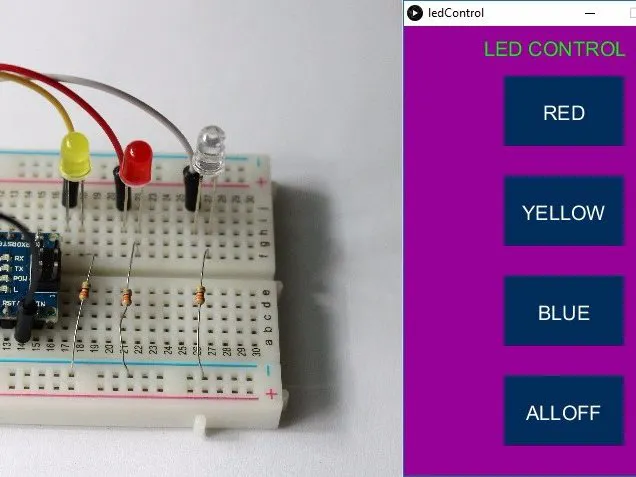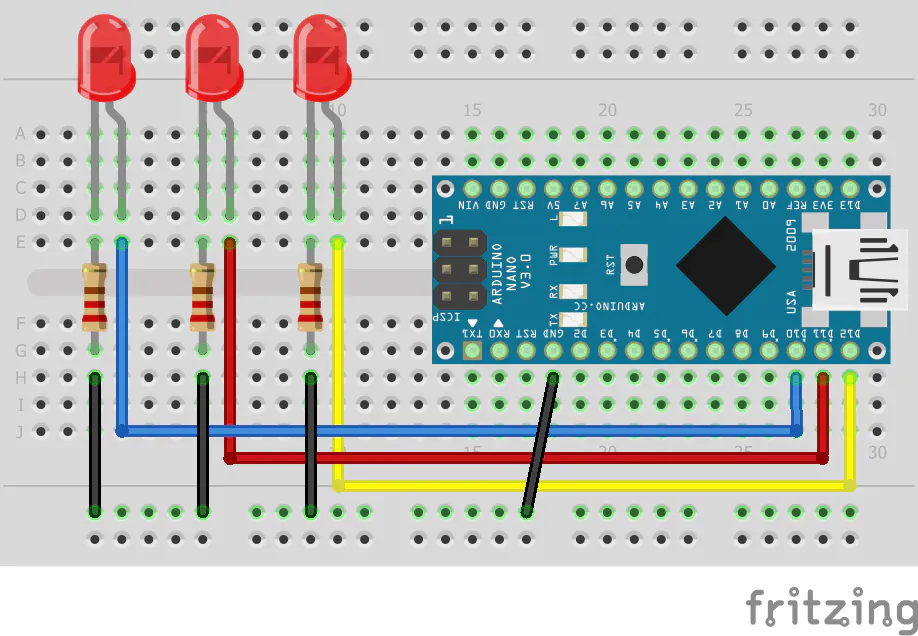# Control Arduino Using GUI (Arduino + Processing)

Very easy and interesting project to create GUI (i.e. Buttons, Sliders, Knobs and many more) to control Arduino

BeginnerFull instructions provided30 minutes79,930## Things used in this project

### Hardware components

 Arduino
×1×1
 LED
×3
 100 Ohm Resistor
×1
 Jumper wires
×4

### Software apps and online services

 Arduino IDE
 Processing

## Schematics

### Circuit## Code

### Arduino Sketch

Arduino
```void setup() {

pinMode(10, OUTPUT);   //set pin as output , blue led
pinMode(11, OUTPUT);   //set pin as output , red led
pinMode(12, OUTPUT);   //set pin as output , yellow led

Serial.begin(9600);    //start serial communication @9600 bps
}

void loop(){

if(Serial.available()){  //id data is available to read

if(val == 'r'){       //if r received
digitalWrite(11, HIGH); //turn on red led
}
if(val == 'b'){       //if b received
digitalWrite(10, HIGH); //turn on blue led
}
if(val == 'y'){       //if y received
digitalWrite(12, HIGH); //turn on yellow led
}
if(val == 'f'){       //if f received
digitalWrite(11, LOW); //turn off all led
digitalWrite(12, LOW);
digitalWrite(10, LOW);
}
}
}
```

### Processing sketch

Processing
```import controlP5.*; //import ControlP5 library
import processing.serial.*;

Serial port;

ControlP5 cp5; //create ControlP5 object
PFont font;

void setup(){ //same as arduino program

size(300, 450);    //window size, (width, height)

printArray(Serial.list());   //prints all available serial ports

port = new Serial(this, "COM3", 9600);  //i have connected arduino to com3, it would be different in linux and mac os

//lets add buton to empty window

cp5 = new ControlP5(this);
font = createFont("calibri light bold", 20);    // custom fonts for buttons and title

cp5.addButton("red")     //"red" is the name of button
.setPosition(100, 50)  //x and y coordinates of upper left corner of button
.setSize(120, 70)      //(width, height)
.setFont(font)
;

cp5.addButton("yellow")     //"yellow" is the name of button
.setPosition(100, 150)  //x and y coordinates of upper left corner of button
.setSize(120, 70)      //(width, height)
.setFont(font)
;

cp5.addButton("blue")     //"blue" is the name of button
.setPosition(100, 250)  //x and y coordinates of upper left corner of button
.setSize(120, 70)      //(width, height)
.setFont(font)
;

cp5.addButton("alloff")     //"alloff" is the name of button
.setPosition(100, 350)  //x and y coordinates of upper left corner of button
.setSize(120, 70)      //(width, height)
.setFont(font)
;
}

void draw(){  //same as loop in arduino

background(150, 0 , 150); // background color of window (r, g, b) or (0 to 255)

//lets give title to our window
fill(0, 255, 0);               //text color (r, g, b)
textFont(font);
text("LED CONTROL", 80, 30);  // ("text", x coordinate, y coordinat)
}

//lets add some functions to our buttons
//so whe you press any button, it sends perticular char over serial port

void red(){
port.write('r');
}

void yellow(){
port.write('y');
}

void blue(){
port.write('b');
}

void alloff(){
port.write('f');
}
```

## Credits

### Hardik Rathod

1 project • 31 followers